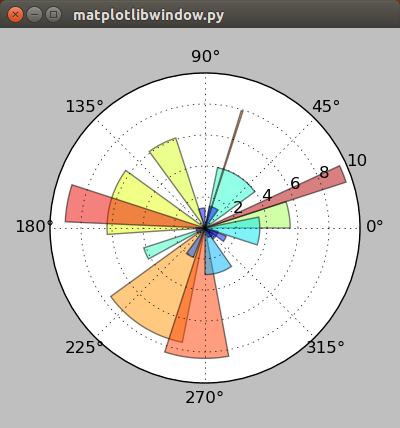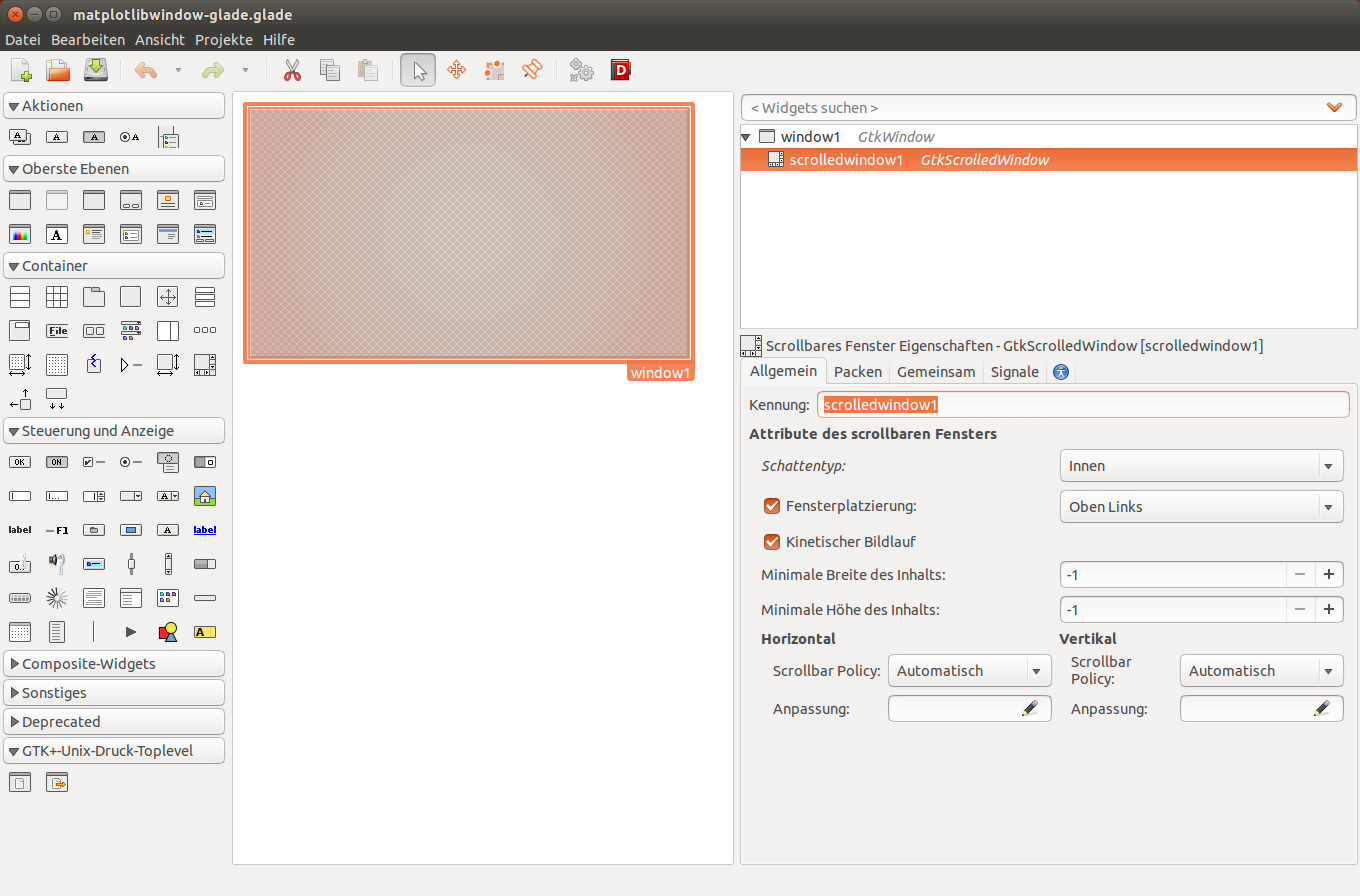# 1. Hello plot!¶

The first chapter will explain how to open an empty GTK3-window and then how to embed Matplotlib into it.

For small applications the GTK3-code can be easily integrated into the Python-code. Building the interface with Glade is a little more complicated in the beginning. With increasing size though, the usage of Glade will become more useful.

## 1.1. Empty GTK 3 window¶

#!/usr/bin/python3

from gi.repository import Gtk

myfirstwindow = Gtk.Window()

myfirstwindow.connect("delete-event", Gtk.main_quit)
myfirstwindow.show_all()
Gtk.main()


These are all the lines that are required for a fully functional window. This is what they do:

The first line helps Unix operating systems to recognize the file format of a file. In this case we wan’t the operating system to know that the file should be excecuted with Python 3.x:

#!/usr/bin/python3


Then the program needs to import the gui-framework (or gui-toolkit). Older Gtk 2.x applications used (import gtk), but for Python 3 and Gtk 3.x applications (i.e. this tutorial) we need:

from gi.repository import Gtk


We can then define an object for a Gtk.Window(), which can have any name:

myfirstwindow = Gtk.Window()


Next the we have to connect our program with the quit-button (x-button) of the window. Otherwise closing the window will not terminate the application:

myfirstwindow.connect("delete-event", Gtk.main_quit)


The next line ensures that the program window is shown. Excluding this line will mean that the program start, but no window is displayed:

myfirstwindow.show_all()


The last line starts the main program loop with all functions. Without this line no loop is started and the program will not do anything:

Gtk.main()


### 1.1.1. Empty window with Glade¶

Opening an empty window with Glade takes a little more effort. First we need to open the Glade interface designer. Then drag a GtkWindow into the workspace. By default the window will be named “window1”, which we can keep. Then we have to set a signal for that window, so we can later close it. The signal we need is “GtkWidget –> destroy” and in the column process we can set “on_window1_destroy”. This will also be the name of the function in the Python code.The steps in Glade are: Create a GtkWindow, then open the Signals tab and enter “on_window1_destroy” for “GtkWidget –> destroy”.

This is all we need for an empty (and closable) window. Then we can save the file with the extension ”.glade”. The finished XML-code of that file looks like this and should be fairly easy to understand:

<?xml version="1.0" encoding="UTF-8"?>
<!-- Generated with glade 3.16.1 -->
<interface>
<requires lib="gtk+" version="3.10"/>
<object class="GtkWindow" id="window1">
<property name="can_focus">False</property>
<signal name="destroy" handler="on_window1_destroy" swapped="no"/>
<child>
<placeholder/>
</child>
</object>
</interface>


Now we have to create a separate file to hold the python code that will call the Glade-file. The finished code looks like this:

#!/usr/bin/python3

from gi.repository import Gtk

class Signals:
def on_window1_destroy(self, widget):
Gtk.main_quit()

builder = Gtk.Builder()
builder.connect_signals(Signals())

myfirstwindow = builder.get_object('window1')

myfirstwindow.show_all()
Gtk.main()


In comparison to the previous approach a few lines of code have changed. First we call the Gtk.Builder() function:

builder = Gtk.Builder()


Then we use the Gtk.Builder() to add the objects from the Glade-file. In the bracket we first need to specify the Glade-file, and then a list of objects even if we just want to call one object (Thankyou errol from http://www.gtkforums.com for this tip):

builder.add_objects_from_file('empty-window-glade.glade', ('window1', '') )


Next the builder needs to connect the signals that we defined in the Glade file. The easiest way of doing this is to place the Signals in their own Class. We only defined one signal in Glade which was “on_window1_destroy”:

builder.connect_signals(Signals())

class Signals:
def on_window1_destroy(self, widget):
Gtk.main_quit()


The last two lines of the program are the same as for the previous example.

## 1.2. Embedding Matplotlib¶

Now that we have an empty window we will learn how to place Matplotlib into it. The main differences are that we need to import Matplotlib-specific packages, insert our Matplotlib-code and place the resulting FigureCanvas in a Gtk.ScrolledWindow (which is a child-element of the Gtk.Window).

We will look at an example that will produce a random radial plot on each application start (adapted from http://matplotlib.org/dev/examples/pie_and_polar_charts/polar_bar_demo.html). The finished Python-code is:

#!/usr/bin/python3

from gi.repository import Gtk

from matplotlib.figure import Figure
from numpy import arange, pi, random, linspace
import matplotlib.cm as cm
#Possibly this rendering backend is broken currently
#from matplotlib.backends.backend_gtk3agg import FigureCanvasGTK3Agg as FigureCanvas
from matplotlib.backends.backend_gtk3cairo import FigureCanvasGTK3Cairo as FigureCanvas

myfirstwindow = Gtk.Window()
myfirstwindow.connect("delete-event", Gtk.main_quit)
myfirstwindow.set_default_size(400, 400)

fig = Figure(figsize=(5,5), dpi=100)

N = 20
theta = linspace(0.0, 2 * pi, N, endpoint=False)
width = pi / 4 * random.rand(N)

bars = ax.bar(theta, radii, width=width, bottom=0.0)

for r, bar in zip(radii, bars):
bar.set_facecolor(cm.jet(r / 10.))
bar.set_alpha(0.5)

ax.plot()

sw = Gtk.ScrolledWindow()

canvas = FigureCanvas(fig)
canvas.set_size_request(400,400)

myfirstwindow.show_all()
Gtk.main()The first window with an embedded Matplotlib-graph as it renders in Ubuntu 14.04.

As you probably noticed we imported a few more modules. The module matplotlib.figure is required to render the graph. We need some functions from NumPy for evenly dividing an interval (numpy.arange), the value for the constant pi (numpy.pi), a function for random numbers (numpy.random) and a function that returns evenly spaced numbers in an interval (numpy.linspace). We also need the colormap function (matplotlib.cm). The container in which the graph is rendered is the FigureCanvasGTK3Agg or FigureCanvasGTK3Cairo.

from matplotlib.figure import Figure
from numpy import arange, pi, random, linspace
import matplotlib.cm as cm
#Possibly this rendering backend is broken currently
#from matplotlib.backends.backend_gtk3agg import FigureCanvasGTK3Agg as FigureCanvas
from matplotlib.backends.backend_gtk3cairo import FigureCanvasGTK3Cairo as FigureCanvas


In order to give the plot a sufficient default size we add this line:

myfirstwindow.set_default_size(400, 400)


Next we create an instance of a matplotlib.figure and define its size and resolution. The resolution is a fixed value, which means that your plots will probably not look the same on newer display with very high pixel-densities. 100 dpi (“dots per inch”, which is a very annoying non-metric unit) works well for regular screens which is about 40 pixels per cm.

fig = Figure(figsize=(5,5), dpi=100)


Then we add a subplot to the plot (This sounds a little weird for only one graph, but you can acutally add many subplots to one plot). 111 means that we have a 1 x 1 grid and are putting the subplot in the 1st cell. Because we want to create a polar plot, we have to set “projection=’polar’”.

ax = fig.add_subplot(111, projection='polar')


Next we will define the number of intervals, and divide a full circle (in radian-units: 2*pi) by that number. Then we create random arrays for 20 radiis and 20 widths for the histogram bars. You can test if the program uses an array by trying “radii = [1,2,3,4,5,6,7,8,9,10,11,12,13,14,15,16,17,18,19,20]” to create bars that have radii from 1 to 20.

N = 20
theta = linspace(0.0, 2 * pi, N, endpoint=False)
width = pi / 4 * random.rand(N)


Then we assign the newly created bars to our plot and store them in a variable:

bars = ax.bar(theta, radii, width=width, bottom=0.0)


We can use this variable “bars” to customize the plot. We will do this for the color in the next piece of code. This algorithm assigns each radius a different color using the “cm.jet” color scheme (See Matplotlib: Colormaps reference for more color maps). Additionaly the alpha of each histogram-bar is set to “0.5”.

for r, bar in zip(radii, bars):
bar.set_facecolor(cm.jet(r / 10.))
bar.set_alpha(0.5)


In the next step we plot the generated graph:

ax.plot()


Then we just have to create a GtkScrolledWindow, and add it to our GtkWindow.

sw = Gtk.ScrolledWindow()


Finally we can create an instance of a FigureCanvasGTK3Agg or FigureCanvasGTK3Cairo with our figure included in it. Then we set the size of the canvas and add embed it into the GtkScrolledWindow.

canvas = FigureCanvas(fig)
canvas.set_size_request(400,400)


### 1.2.1. Embedding Matplotlib with Glade¶

Recreating the previous example with Glade requires just some minor changes to the Glade-file and a slightly different Python-3-code.

First we need to add a GtkScrolledWindow to our empty window. Although the name has “window” in it, it actually is more like a canvas for other widgets. In the Python code we will place in the next step a FigureCanvas into the GtkScrolledWindow. In order to give the plot more space, we can also set the default width and height of “window1” to 400 px each.Starting with the previous example all we need to add is a GtkScrolledWindow.

The XML-code of the Glade-file after the modifications looks like this:

<?xml version="1.0" encoding="UTF-8"?>
<!-- Generated with glade 3.16.1 -->
<interface>
<requires lib="gtk+" version="3.10"/>
<object class="GtkWindow" id="window1">
<property name="can_focus">False</property>
<property name="default_width">400</property>
<property name="default_height">400</property>
<signal name="destroy" handler="on_window1_destroy" swapped="no"/>
<child>
<object class="GtkScrolledWindow" id="scrolledwindow1">
<property name="visible">True</property>
<property name="can_focus">True</property>
<child>
<placeholder/>
</child>
</object>
</child>
</object>
</interface>


Starting with the code from the previous examples we only have to make slight changes to port this example. This is the final result:

#!/usr/bin/python3

from gi.repository import Gtk

from matplotlib.figure import Figure
from numpy import arange, pi, random, linspace
import matplotlib.cm as cm
#Possibly this rendering backend is broken currently
#from matplotlib.backends.backend_gtk3agg import FigureCanvasGTK3Agg as FigureCanvas
from matplotlib.backends.backend_gtk3cairo import FigureCanvasGTK3Cairo as FigureCanvas

class Signals:
def on_window1_destroy(self, widget):
Gtk.main_quit()

builder = Gtk.Builder()
builder.connect_signals(Signals())

myfirstwindow = builder.get_object('window1')
sw = builder.get_object('scrolledwindow1')

fig = Figure(figsize=(5,5), dpi=100)

N = 20
theta = linspace(0.0, 2 * pi, N, endpoint=False)
width = pi / 4 * random.rand(N)

bars = ax.bar(theta, radii, width=width, bottom=0.0)

for r, bar in zip(radii, bars):
bar.set_facecolor(cm.jet(r / 10.))
bar.set_alpha(0.5)

ax.plot()

canvas = FigureCanvas(fig)

myfirstwindow = builder.get_object('window1')